# Data Wrangling in R Programming – Working with Tibbles

R is a robust language used by Analysts, Data Scientists, and Business users to perform various tasks such as statistical analysis, visualizations, and developing statistical software in multiple fields.

Data Wrangling is a process reimaging the raw data to a more structured format, which will help to get better insights and make better decisions from the data.

#### What are Tibbles?

Tibbles are the core data structure of the `tidyverse` and is used to facilitate the display and analysis of information in a tidy format. Tibbles is a new form of data frame where data frames are the most common data structures used to store data sets in R.

#### Advantages of Tibbles over data frames

• All Tidyverse packages support Tibbles.
• Tibbles print in a much cleaner format than data frames.
• A data frame often converts character strings to factor and analysts often have to override the setting while Tibbles doesn’t try to make this conversion automatically.

#### Different ways to create Tibbles

• as_tibble():
The first function is as tibble function. This function is used to create a tibble from an existing data frame.

Syntax:
as_tibble(x, validate = NULL, …)

x is either a data frame, matrix, or list.

• tibble():
The second way is to use a `tibble()` function, which is used to create a tibble from scratch.

Syntax:
tibble(s…, rows = NULL)

s represents a set of name-value pairs.

• Import():
Finally, you can use the tidyverse’s data import packages to create Tibbles from external data sources such as databases or CSV files.

Syntax: import(pkgname …)

• library():
The `library()` function is used to load the namespace of the package.

Syntax:
library(package, help, pos = 2, lib.loc = NULL)

`Note: To find more about the functions in R, type ? followed by function name. Eg: ?tibble.`

Let us see some examples of how to use the above functions using Rstudio IDE. We will be using a builtin dataset (CO2) Carbon Dioxide Uptake in Grass Plants to create a tibble.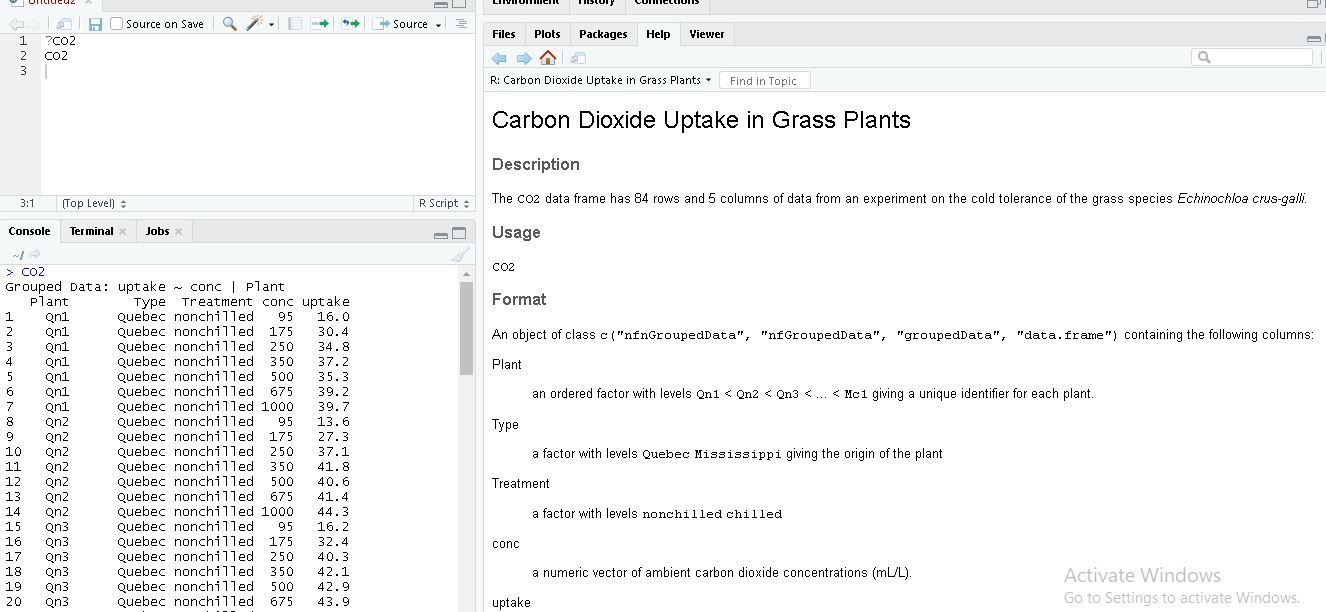This dataset consists of several variables, such as plant, type, treatment, concentration, and uptake. It is difficult to work with this type of information, so let us convert this information into a tibble. Let us create a tibble named sample_tibble from CO2 dataset using as_tibble() function.

#### Example of as_tibble()

Here we are converting a data frame (CO2) into tibble using `as_tibble()` function. It requires you to install tidyverse package in Rstudio.

 `library(tidyverse)         ``# loading tidyverse package       ` `sample_tibble <``-` `as_tibble(CO2)   ``# creating a tibble named sample_tibble ` `print``(sample_tibble)        `

Output: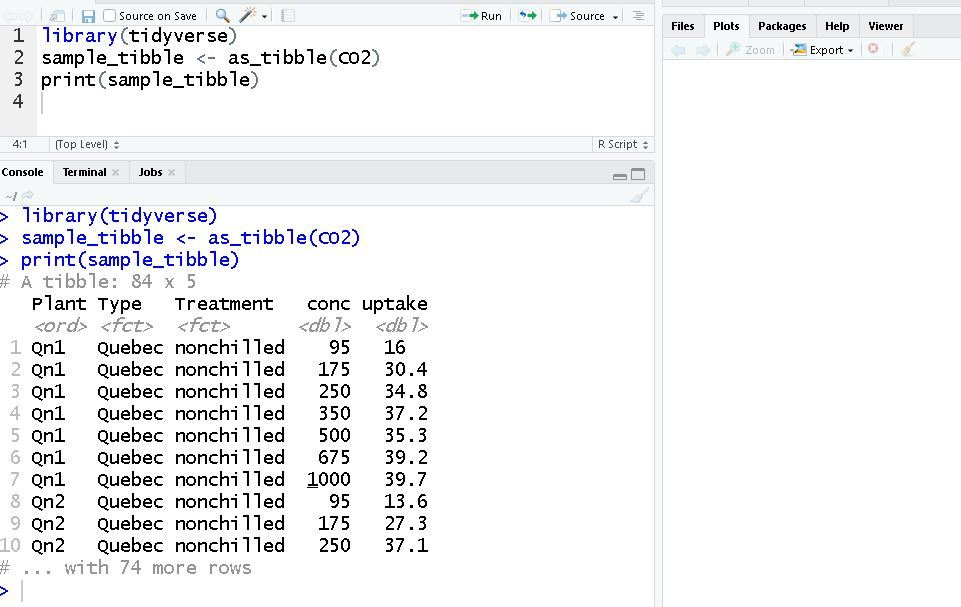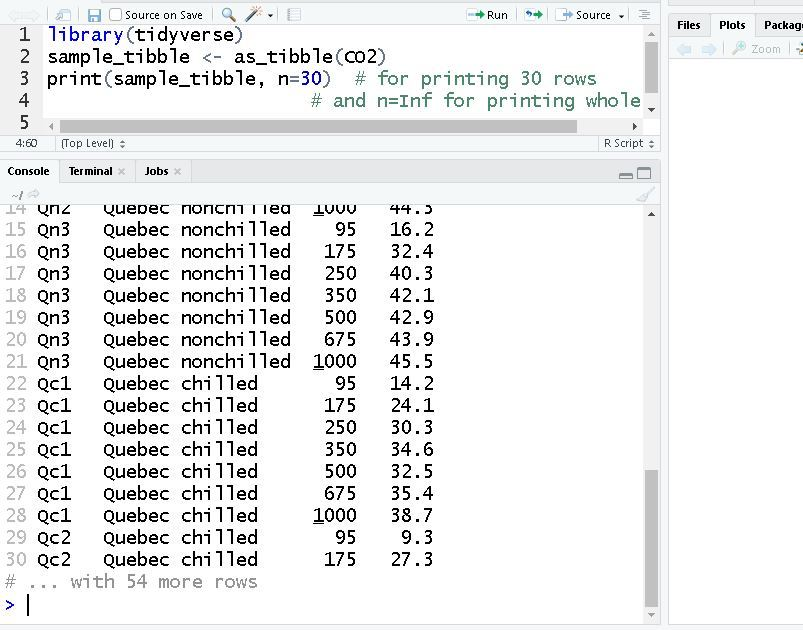#### Example of tibble()

The second Method was to create a tibble from scratch using `tibble()` function so we will create few vectors such as name, marks_in_Math, marks_in_Java, Fav_color etc and pass them to `tibble()` function which converts them into tibble.

 `library(tidyverse) ` `name <``-` `c(``"surya"``, ``"sai"``, ``"Nihith"``, ``"prakash"``, ``"vikas"``, ``"mayur"``) ` `marks_in_Math <``-` `c(``91``, ``85``, ``92``, ``89``, ``90``, ``93``) ` `marks_in_Java <``-` `c(``89``, ``91``, ``88``, ``91``, ``89``, ``87``) ` `Fav_color <``-` `c(``"Pink"``, ``"Red"``, ``"Yellow"``, ``"Green"``, ``"White"``, ``"Blue"``) ` `students <``-` `tibble(name, marks_in_Math, marks_in_Java, Fav_color) ` `print``(students) `

Output: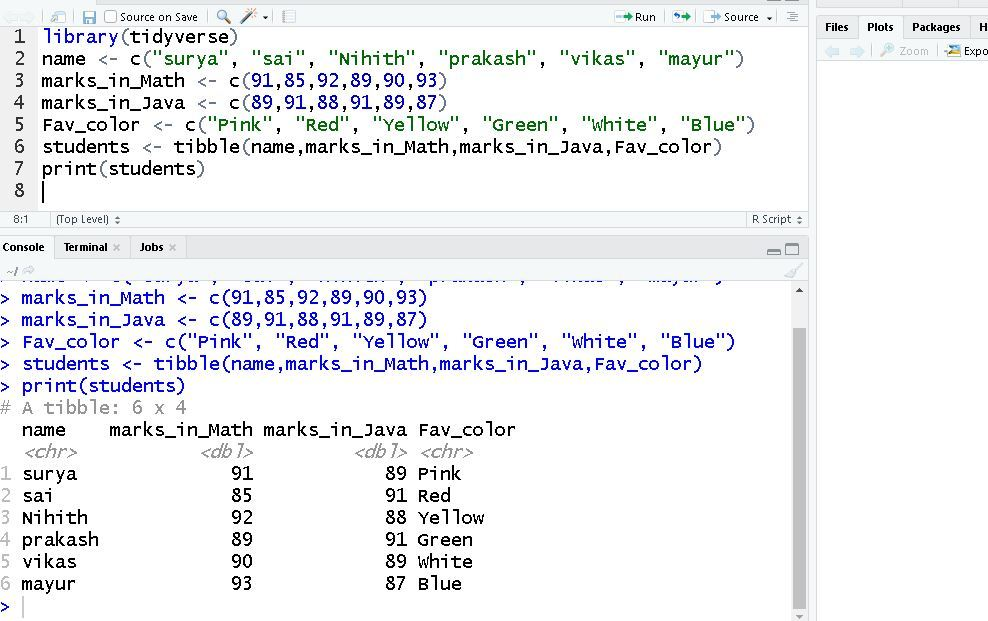### Subsetting tibbles

Data analysts often extract a single variable from a tibble for further use in their analysis, which is called subsetting. When we try to subset a tibble, we extract a single variable from the Tibble in vector form. We can do this by using a few special operators.

• \$ Operator
• [[]] Operator

#### \$ Operator

The first way we can extract a variable from Tibble is by using a dollar(\$) sign, operator. To do this, we will be creating a tibble from scratch using a `tibble()` function.

 `library(tidyverse) ` `name <``-` `c(``"surya"``, ``"sai"``, ``"Nihith"``, ``"prakash"``, ``"vikas"``, ``"mayur"``) ` `marks_in_Math <``-` `c(``91``, ``90``, ``91``, ``85``, ``90``, ``92``) ` `marks_in_Java <``-` `c(``91``, ``91``, ``92``, ``91``, ``89``, ``93``) ` `Fav_color <``-` `c(``"Pink"``, ``"Red"``, ``"Yellow"``, ``"Green"``, ``"White"``, ``"Blue"``) ` ` `  `students <``-` `tibble(name, marks_in_Math, marks_in_Java, Fav_color) ` `students\$Fav_color ` `students\$marks_in_Math `

Output: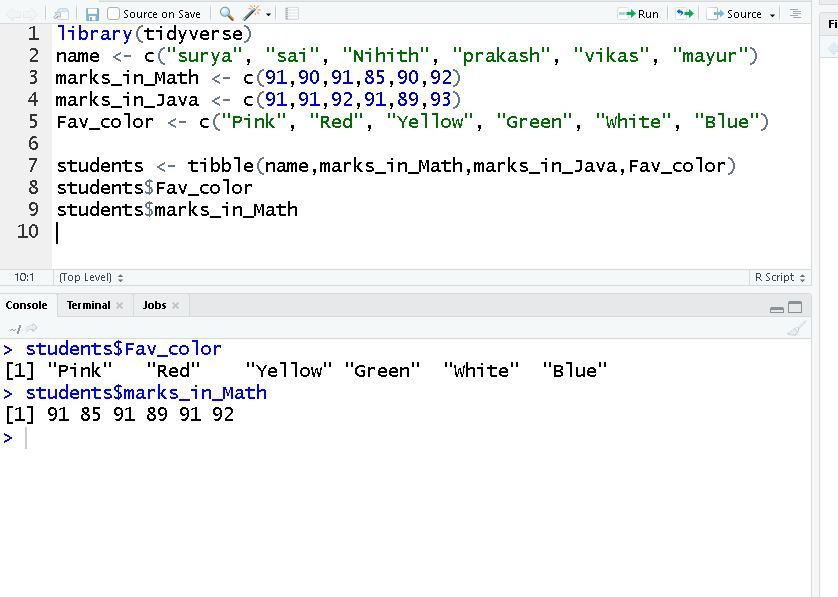#### [[]] Operator

The second way you can access a single variable from Tibble is by using square braces([[]]). We will use the same tibble created previously.

 `library(tidyverse) ` `name <``-` `c(``"surya"``, ``"sai"``, ``"Nihith"``, ``"prakash"``, ``"vikas"``, ``"mayur"``) ` `marks_in_Math <``-` `c(``91``, ``90``, ``91``, ``85``, ``90``, ``92``) ` `marks_in_Java <``-` `c(``91``, ``91``, ``92``, ``91``, ``89``, ``93``) ` `Fav_color <``-` `c(``"Pink"``, ``"Red"``, ``"Yellow"``, ``"Green"``, ``"White"``, ``"Blue"``) ` ` `  `students <``-` `tibble(name, marks_in_Math, marks_in_Java, Fav_color) ` `students\$Fav_color ` `students[[``"name"``]] ` `students[[``"marks_in_Math"``]] `

Output: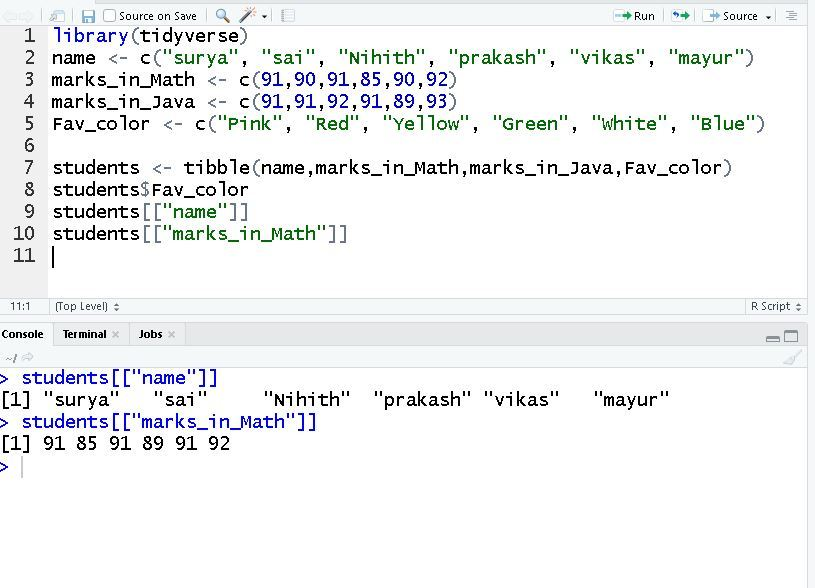#### Filtering Tibbles

Filtering provides a way to help reduce the number of rows in your tibble. When performing filtering, we can specify conditions or specific criteria that are used to reduce the number of rows in the dataset.

filter() Function:

Syntax: filter(data, conditions)

The data represents the Tibble name, and conditions are used to specify an expression that returns a logical value. We will be using the student’s Tibble, which we created in the above example.

 `library(tidyverse) ` `name <``-` `c(``"surya"``, ``"sai"``, ``"Nihith"``, ``"prakash"``, ``"vikas"``, ``"mayur"``) ` `marks_in_Math <``-` `c(``91``, ``90``, ``91``, ``85``, ``90``, ``92``) ` `marks_in_Java <``-` `c(``91``, ``91``, ``92``, ``91``, ``89``, ``93``) ` `Fav_color <``-` `c(``"Pink"``, ``"Red"``, ``"Yellow"``, ``"Green"``, ``"White"``, ``"Blue"``) ` ` `  `students <``-` `tibble(name, marks_in_Math, marks_in_Java, Fav_color) ` `filter_students ``=``90``) ` `print``(filter_students) `

Output: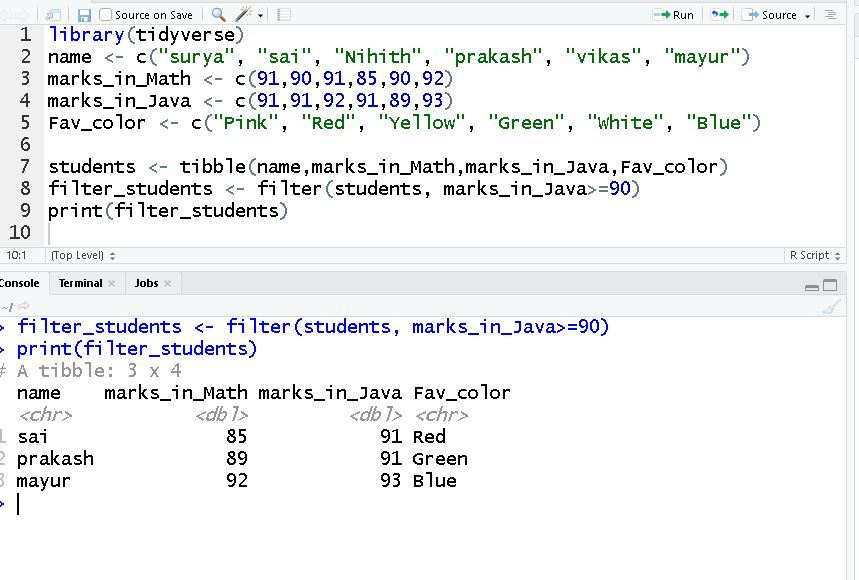My Personal Notes arrow_drop_upIf you like GeeksforGeeks and would like to contribute, you can also write an article using contribute.geeksforgeeks.org or mail your article to contribute@geeksforgeeks.org. See your article appearing on the GeeksforGeeks main page and help other Geeks.

Please Improve this article if you find anything incorrect by clicking on the "Improve Article" button below.

Article Tags :

1

Please write to us at contribute@geeksforgeeks.org to report any issue with the above content.